Jr Math section of MathBitsNotebook.comFractions and DecimalsTopics Standards: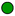NGMS-6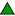CCSS 6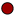NGMS-7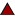CCSS 7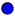NGMS-8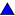CCSS 8

*
Fraction Basics (visualizing, number line, decompose, improper, mixed number)
Review
*
Comparing Fractions (equivalent, reducing, < , > , ordering)
Review
*
Adding Fractions (rule, same (different) denominators, mixed numbers, number line)
Review
*
Subtracting Fractions (rule, same (different) denom., mixed numbers, number line)
Review
*
Multiplying Fractions (rule, examples, visualize, number line)
Review
*
Dividing Fractions (rule, examples, visualize, number line, origin of rule)*
Complex Fractions (fractions within fractions)Fraction Properties (reference chart)
ChartPRACTICE: • Practice with Fraction Basics Review • Practice Comparing Fractions• Review • Practice Multiplying and Dividing Fractions•• Practice with Applications of Fractions• Practice with Complex Fractions• Vocabulary - Word Guess Game (Jr level topical vocabulary/content game - fractions)• Practice with Decimals and Fractions